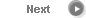#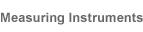# Precise Color Communication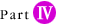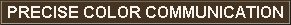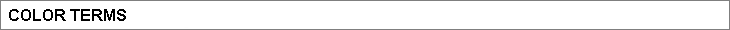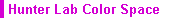The Hunter Lab space was developed in 1948 by R. S. Hunter as a uniform color space which could be read directly from a photoelectric colorimeter (tristimulus method). Values in this space are defined by the following formulas: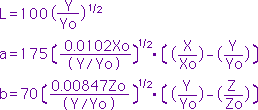where X, Y, Z: Tristimulus values of the specimen (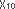,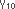,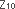tristimulus values can also be used.) X0, Y0, Z0: Tristimulus values of the perfect reflecting diffuser For the 2˚ Standard Observer and Standard Illuminant C, the above equations would become: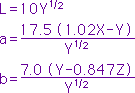Color difference ∆EH in the Hunter Lab color space, which indicates the degree of color difference but not the direction, is defined by the following equation: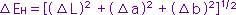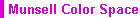The Munsell color system consists of a series of color charts which are intended to be used for visual comparison with the specimen. Colors are defined in terms of the Munsell Hue (H; indicates hue), Munsell Value (V; indicates lightness), and Munsell Chroma (C; indicates saturation) and are written as H V/C. For example, for the color with H=5.0R, V=4.0, and C=14.0, the Munsell notation would be: 5.0R 4.0/14.0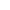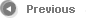6/12